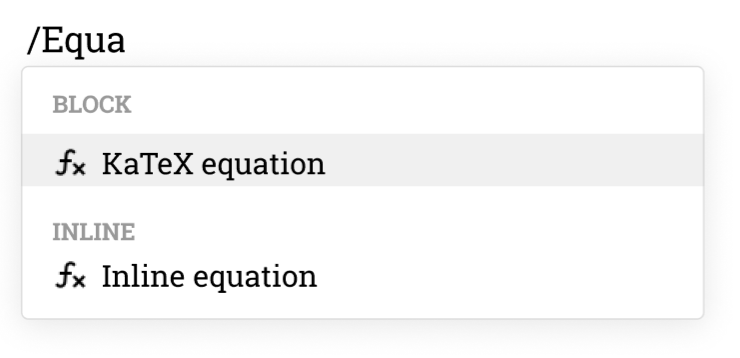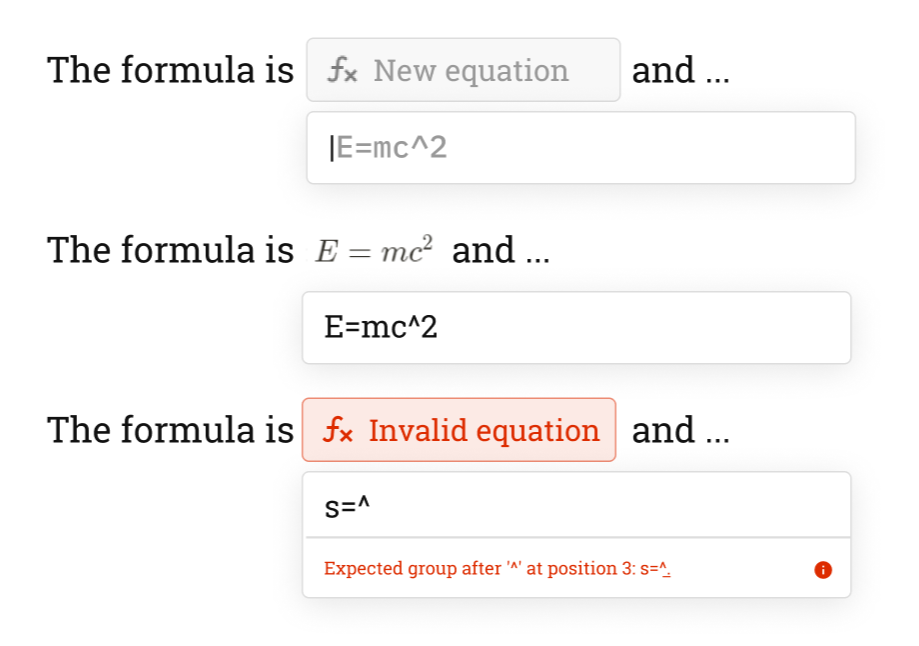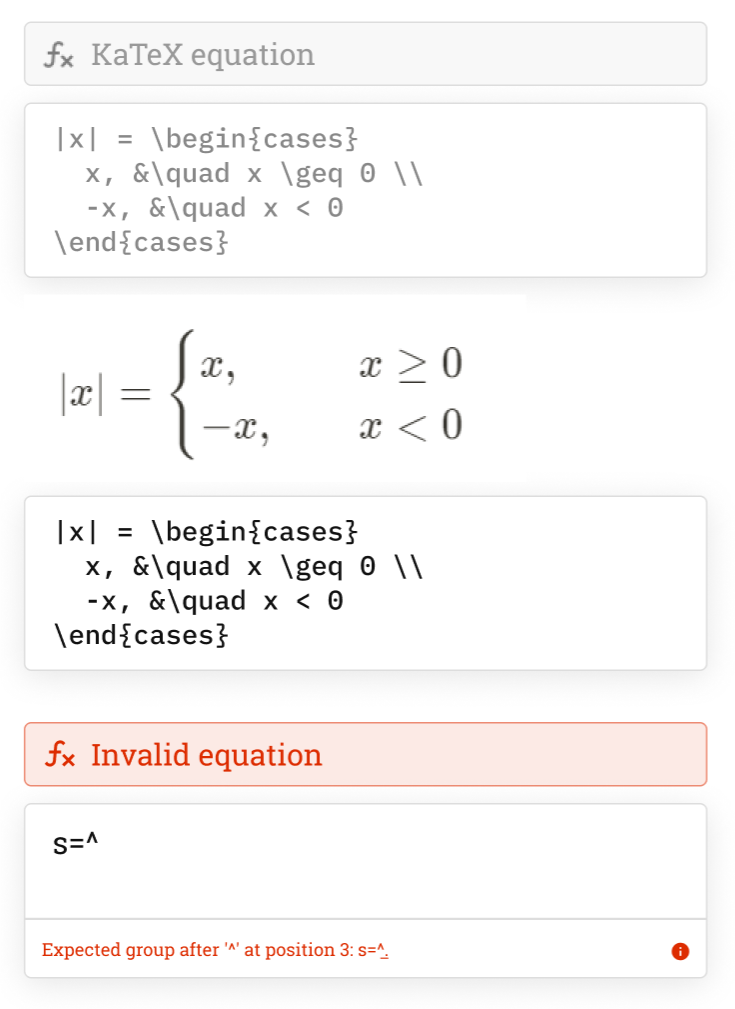# Colabra & LaTeX

Insert math equations into your electronic lab notebook.

## Features

### Inline equations

Insert short math equations inline.#### How to use

Type /Inline equation, enter your KaTeX equation, and press Enter.

### Block equations

Insert complex KaTeX equations in your experimental notes.#### How to use

Type /KaTeX equation, enter your KaTeX code, and press Enter.